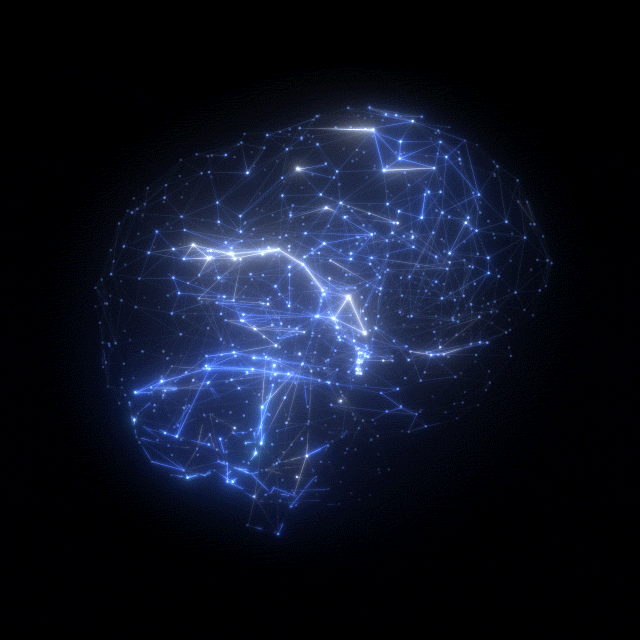# Getting started with 🧠Pytorch: Basic functions 💬## But what is Pytorch exactly? 🙄

PyTorch is an open-source machine learning library based on the Torch library, used for applications such as computer vision and natural language processing, primarily developed by Facebook’s AI Research lab (FAIR). It is free and open-source software released under the Modified BSD license. Although the Python interface is more polished and the primary focus of development, PyTorch also has a C++ interface. A number of pieces of Deep Learning software are built on top of PyTorch, including Tesla Autopilot, Uber’s Pyro, HuggingFace’s Transformers, etc.

## Features of Pytorch 🔤

• Tensor computing (like NumPy) with strong acceleration via graphics processing units (GPU)
• Deep neural networks built on a tape-based automatic differentiation system

## A short introduction about the chosen functions. ⛑️These are the 5 basic functions(+1 bonus) that you should know before diving deep into the Pytorch world.

function 1- torch.as_strided
function 2- torch.rands
function 3- torch.size
function 4- torch.ones
function 6- torch.full

## ✍🏽 Function 1 : as_strided

as_strided function displays the tensor in a different shape and with values based on the specified parameters. it takes 3 parameters. the tensor itself , the shape in which to be displayed and the difference in position of the element adjacent to it in the columnwise and also row wise as a tuple.

import torch
# as_stride function
x = torch.tensor([[1.,2,3],[4.,5,6],[7.,8,9]])
y = torch.as_strided(x,(3,2),(3,1))
y
tensor([[1., 2.],
[4., 5.],
[7., 8.]])

## ✍🏽 Function 2: cat

Cat function concatenates tensor x 3 times and create new tensor.

x = torch.randn(2, 4)
torch.cat((x, x, x), 0)
tensor([[ 0.8192,  2.0575, -0.3061, -1.2949],
[ 0.5110,  0.9729, -0.9524, -1.7303],
[ 0.8192,  2.0575, -0.3061, -1.2949],
[ 0.5110,  0.9729, -0.9524, -1.7303],
[ 0.8192,  2.0575, -0.3061, -1.2949],
[ 0.5110,  0.9729, -0.9524, -1.7303]])

## ✍🏽 Function 3: size

b is a tensor created from a.view(15) that reshaped the 2D tensor into a 1D tensor. To create tensor c from a using tensor.view we passed the first dimension as -1 and second dimension as 3. The first dimension is inferred from the shape of original tensor.

 a = torch.rand(3,5)
print(a.size())
b = a.view(15)
print(b.size())
c = a.view(-1,3)
print(c.size())
torch.Size([3, 5])
torch.Size()
torch.Size([5, 3])

## ✍🏽 Function 4: ones

This function returns a tensor filled with the value 1, with the shape defined by the variable argument size.

torch.ones(3,3)
tensor([[1., 1., 1.],
[1., 1., 1.],
[1., 1., 1.]])

## ✍🏽 Function 5: full

The full function generates a tensor of a specified size with all elements being equal to the number specified.

# the full function

a = torch.full((2,3),5.)
a
tensor([[5., 5., 5.],
[5., 5., 5.]])

## ✍🏽 Bonus Function: arange

The arange function generates a tensor that contains elements of value starting from the first parameter to second paramenter-1 . with the step as the third parameter .

# the arrange function

a = torch.arange(0,10,2)
a
tensor([0, 2, 4, 6, 8])

## Important! 🖼️This is the link to my Jovian notebook where I have used all these functions. Feel free to explore! Here you go! 💫

## Pytorch vs Tensorflow 🤨

Both TensorFlow and PyTorch have their advantages as starting platforms to get into neural network programming. Traditionally, researchers and Python enthusiasts have preferred PyTorch, while TensorFlow has long been the favored option for building large scale deep learning models for use in production.

## Closing and Summary 🌤️We learnt that PyTorch is a Python package that provides two high-level features:

1. Tensor computation (like NumPy) with strong GPU acceleration.
2. Deep neural networks built on a tape-based autograd system. You can reuse your favorite Python packages such as NumPy, SciPy and Cython to extend PyTorch when needed. PyTorch is a community driven project with several skillful engineers and researchers contributing to it.

## Resources 📕Let’s Start! Check out the original post on Medium.